If you weigh 685 NN on the earth, what would be your weight on the surface of a neutron star that has the same mass as our sun and a diamete

Question

If you weigh 685 NN on the earth, what would be your weight on the surface of a neutron star that has the same mass as our sun and a diameter of 25.0 kmkm ? Take the mass of the sun to be msmsm_s = 1.99×1030 kgkg , the gravitational constant to be GGG = 6.67×10−11 N⋅m2/kg2N⋅m2/kg2 , and the free-fall acceleration at the earth’s surface to be ggg = 9.8 m/s2m/s2 .

in progress 0
6 months 2021-08-04T11:39:28+00:00 2 Answers 21 views 0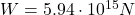Explanation:

To calculate the weight on the surface of a neutron star we can use the following equation: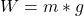Where:

W: is the weight of the person

m: is the mass of the person

g: is the gravity of the neutron star

Hence, first we need to find m and g. The mass is equal to: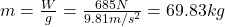Now, the gravity of the neutron star can be found using the followig equation: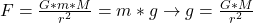Where:

G: is the gravitational constant = 6.67×10⁻¹¹ m³ kg⁻¹ s⁻²

M: is the mass of the neutron star = 1.99×10³⁰ kg

r : is the distance between the person and the surface of the neutron star = 25/2 = 12.5 km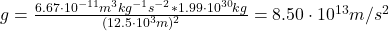Now, we can find the weight on the surface of the neutron star: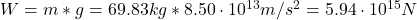I hope it helps you!

W=5.92*10^13 N

Explanation:

The mass is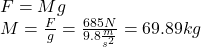Hence, the force in the nuetron star is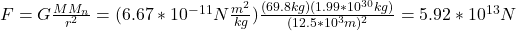Thus, it will be a great weigth!!

Hope this helps